www.batmath.it

### Exercises on matrix addition and multiplication

1. Given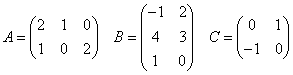, calculate ABC and check the validity of the associative law.
2. Calculate the product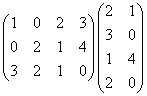3. Given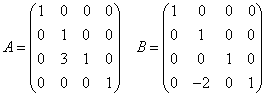, prove that AB = BA.
4. Given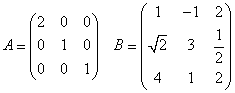, calculate AB and BA.
5. Given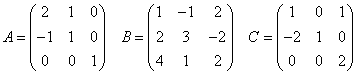, prove that A(B+C)=AB+AC.
first published on march 15 2002 - last updated on september 01 2003